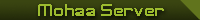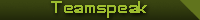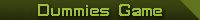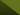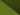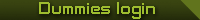(such as player-stats!)

Register at forum..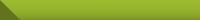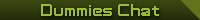Yust a Chatterblock  [View Oldlog]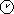_[B.A.D]_Ayreon:First!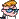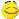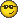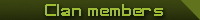Active Clanmembers:
_[B.A.D]_Ayreon (Co CL) WA TL
_[B.A.D]_MI-7 (Co CL) Webmaster
_[B.A.D]_ZoDiAC (Co CL)

Inactive Clanmembers:
_[B.A.D]_Abbath
_[B.A.D]_Bruce
_[B.A.D]_CatScCaNn^ (TO)
_[B.A.D]_Charlie (TO)
_[B.A.D]_Clem
_[B.A.D]_DarkBlue
_[B.A.D]_El Guildo
_[B.A.D]_Geo
_[B.A.D]_Hawk
_[B.A.D]_Infin1ty
_[B.A.D]_J-six
_[B.A.D]_KillingAngel (NL)
_[B.A.D]_mohave
_[B.A.D]_Pieter (TO)
_[B.A.D]_Scorpion
_[B.A.D]_xFighter (TO)
_[B.A.D]_Zly